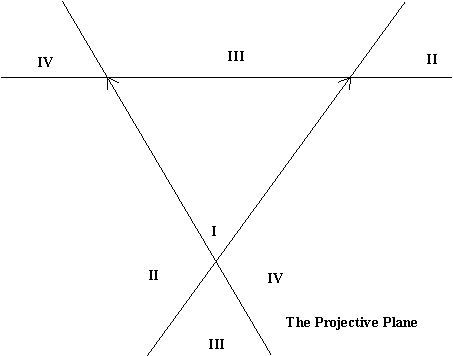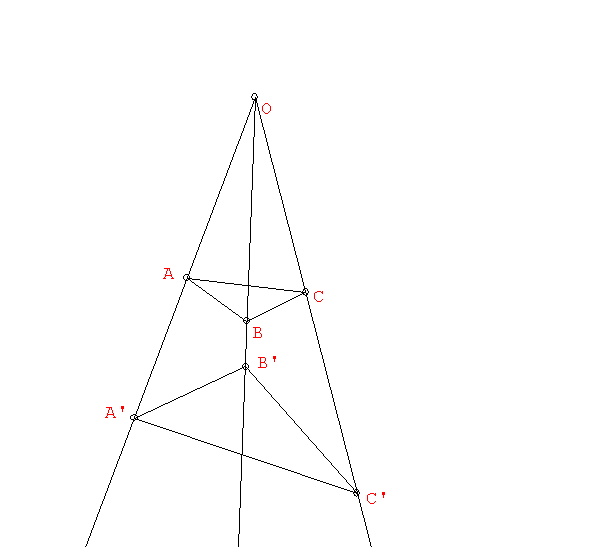Math 105
Tuesday,  May 2

Review from last class:

"Seeing the Infinite"

Seeing the infinite... large.

How to see the Infinite using "Projective geometry".

We will try to understand
• why we see the horizon on a plane....
• why the horizon is a line composed of ideal/infinite points.
• why parallel lines "meet" on the horizon.

Center of projection/ perspective.
[compare with similarity and geometric series].
Correspondences:

Two Line segments of different length:
Different lengths- 1:1 correspondence of points!

Semicircle to line and line to semicircle!
Two points at "infinity"
Circle to line and line to Circle!
One point at "infinity"

How to see the Infinite on a line using Projective geometry.
Line to Circle to Line

Use Rope and lines on floor with an image plane to see parallels meet on an "image" plane ---
where is the horizon?

Perspective between planes in Space!
Source plane ( Flatland)  ----> Image plane (Canvass)

Seeing the horizon on a plane.... a line of ideal/infinite points.

How parallel lines "meet" on the horizon.
Drawing with perspective:
one point- two point (some java examples from UIUC)

## Pictures in perspective:WebMuseum.

Albrecht Durer : St. Jerome dans sa Cellule. (A smaller image )
da Vinci:  Last Supper.
Veronese: The Marriage at Cana
RAPHAEL: School of Athens
Hogarth: Perspective absurdities### Albrecht Dürer's Draughtsman Making a Perspective Drawing of a Woman (1525)Durer: Unterweisung der Messung 1525
from Albrecht Durer’s “Treatise on measurement with compasses and straightedge
(Underweysung der Messung mit dem Zirkel und Richtscheyt, Nuremberg, 1525
What is the Affine Plane? the Projective Plane?How do we see curves in perspective, drawn in the Projective Plane?
Using coordinates to draw in perspective.

Activity: Creating coordinates in the affine plane.
Question: What is on the other side of the horizon?

The affine plane -
The Horizon is a special line- not a part of the plane.
The projective plane - The horizon is just another line- not special!

Activity: Using coordinates to transfer a circle to the affine plane as an ellipse.Equations for conics in coordinate geometry.Activity: Using coordinates to draw in perspective.

What do the conics look like in a perspective drawing or in the projective plane?

Another resource worth looking at :
The Geometry of Perspective Drawing on the Computer

Projective geometry: The study of properties of figures that are related by projections... perspectivities and projectivities.

Examples: The projection of a line is a line. The point of intersection of two lines will project to the point of intersection of the projected lines.

Desargues' Theorem in Space:

We define a perspective relation:
Two points P and P' are perspectively related by the center O if O is on the line PP" . Two triangles ABC and A'B'C' are perspectively related by the center O if O is on the lines AA', BB', and CC'.Another aspect of Projection: Desargues' Theorem in 3-space and the plane.

Some final remarks on the projective plane and visualizing Flatland and Space.:

• What is on the other side of the horizon?
• The projective plane as a surface.
• Visualizing the projective plane on a disc with the boundary identified as the horizon.
• The projective plane as a non-euclidean flatland.

The End!